# OpenAI GPT Models

## Introduction

OpenAI Generative Pre-trained Transformer (GPT) models are a series of transformer architecture based language models trained on a large corpus of text data to generate human-like text developed by OpenAI. Since the rise of transformer model, OpenAI has been continuing on the track of optimizing the GPT models using larger and better datasets, improved neural network modules, human supervision and assistance, and other innovations.

As of 2023, the latest GPT model, ChatGPT, has become the most popular application in the world that helps the users solving problems in different specialized domains. In this article, I would like to discuss the evolution of OpenAI GPT models and their technical details.

## Prerequisites

There are a couple of prerequisites for understanding the GPT evolution, including the transformer model, language modeling, and natural language processing task specific fine-tuning.

### Transformer

The transformer model is the key neural network architecture for the OpenAI GPT models. To learn the transformer model, if you have not done so, please read my previous article “Transformer Explained in One Single Page” for transformer basics and “Transformer Autoregressive Inference Optimization” for in-depth understanding of the transformer decoder autoregressive decoding process.

### Language Modeling

A language model is a probability distribution over sequences of token variables $W = \{ W_1, W_2, W_3, \cdots, W_n \}$, namely, $P(W) = P(W_1, W_2, W_3, \cdots, W_n)$. To optimize such a language model so that the language sampled from the probability distribution is natural to human beings, usually we train the model with the text data that is created by human beings. The optimization for most of the language models is the maximum likelihood estimation with respect to the model parameters given the text training data. Concretely,

\DeclareMathOperator*{\argmin}{argmin} \DeclareMathOperator*{\argmax}{argmax} \begin{aligned} \theta^{\ast} &= \argmax_{\theta} \prod_{i = 1}^{n} P_{\theta}(w_{i}) \\ &= \argmax_{\theta} \sum_{i = 1}^{n} \log P_{\theta}(w_{i}) \\ \end{aligned}

where $\theta$ are the language model paramters, $n$ is the number of sequences from the dataset, $w_{i}$ is the $i$-th sequence from the dataset.

Suppose $W = \{ W_1, W_2, W_3, \cdots, W_n \}$, according to the chain rule,

\begin{aligned} P_{\theta}(W) &= P_{\theta}(W_{1}, W_{2}, W_{3}, \cdots, W_{n}) \\ &= P_{\theta}(W_{1})P_{\theta}(W_{2} \vert W_{1})P_{\theta}(W_{3} \vert W_{1}, W_{2}) \cdots P_{\theta}(W_{n} \vert W_{1}, W_{2}, \cdots, W_{n-1}) \\ &= \prod_{i=1}^{n} P_{\theta}(W_{i} \vert W_{1}, W_{2}, \cdots, W_{i-1}) \end{aligned}

In practice, the language models, such as recurrent neural networks and transformer models, are optimized using the following formula.

\begin{aligned} \theta^{\ast} &= \argmax_{\theta} \sum_{i = 1}^{n} \log P_{\theta}(w_{i}) \\ &= \argmax_{\theta} \sum_{i = 1}^{n} \sum_{j=1}^{m_{i}} \log P_{\theta}(w_{i, j} \vert w_{i, 1}, w_{i, 2}, \cdots, w_{i, j-1}) \\ \end{aligned}

where $m_i$ is the number of tokens in the sequence $w_{i}$, and $w_{i, j}$ is the $j$-th token in the sequence $w_{i}$.

### Natural Language Process Task Specific Fine-Tuning

There were specialized models and datasets developed specifically for certain natural language processing tasks, such as named-entity recognition (NER), machine translation (MT), and question-and-answer (QA). Each data from the dataset consists of a pair of inputs $X$ and outputs $Y$.

The model optimization is a supervised training process that minimizes the expectation of the prediction loss. Concretely,

\begin{aligned} \theta^{\ast} &= \argmin_{\theta} \mathbb{E}_{(X, Y) \sim P_{\text{dataset}}(X, Y)} \left[ L\left(Y, f_{\theta}\left(X\right)\right) \right] \\ &= \argmin_{\theta} \frac{1}{n} \sum_{i = 1}^{n} L\left(y_{i}, f_{\theta}\left(x_{i} \right)\right) \\ \end{aligned}

where $n$ is the number of data from the dataset and $(x_{i}, y_{i})$ is the $i$-th annotated data from the dataset.

## GPT-1

GPT-1 was the first-generation of the GPT models that OpenAI developed for natural language processing tasks in 2018. The major conclusion from the model and its paper “Improving Language Understanding by Generative Pre-Training” were it is no longer necessary to develop specific neural network architectures for specific natural language processing tasks and transfer learning from autoregressive transformer decoder language model pre-trained with large corpus of text data is sufficient for specific natural language processing tasks.

Similar to autoregressive recurrent neural networks which used to be the gold models for language modeling, it’s very straightforward to train an autoregressive transformer decoder using large natural language dataset in a self-supervised fashion. The advantage of transformer for language modeling is that the number of tokens used for training and inference in a transformer model can be much larger. Unlike recurrent neural networks, usually the transformer user does not have to worry about the gradient diminishing effect of backpropagation through time that often happens in the recurrent neural network training, or the model cannot remember the early context that often happens in the recurrent neural network inference, due to there are too many tokens to train or infer (decode). This makes transformer a much better architecture for language model and other natural language processing tasks that require understanding text contexts.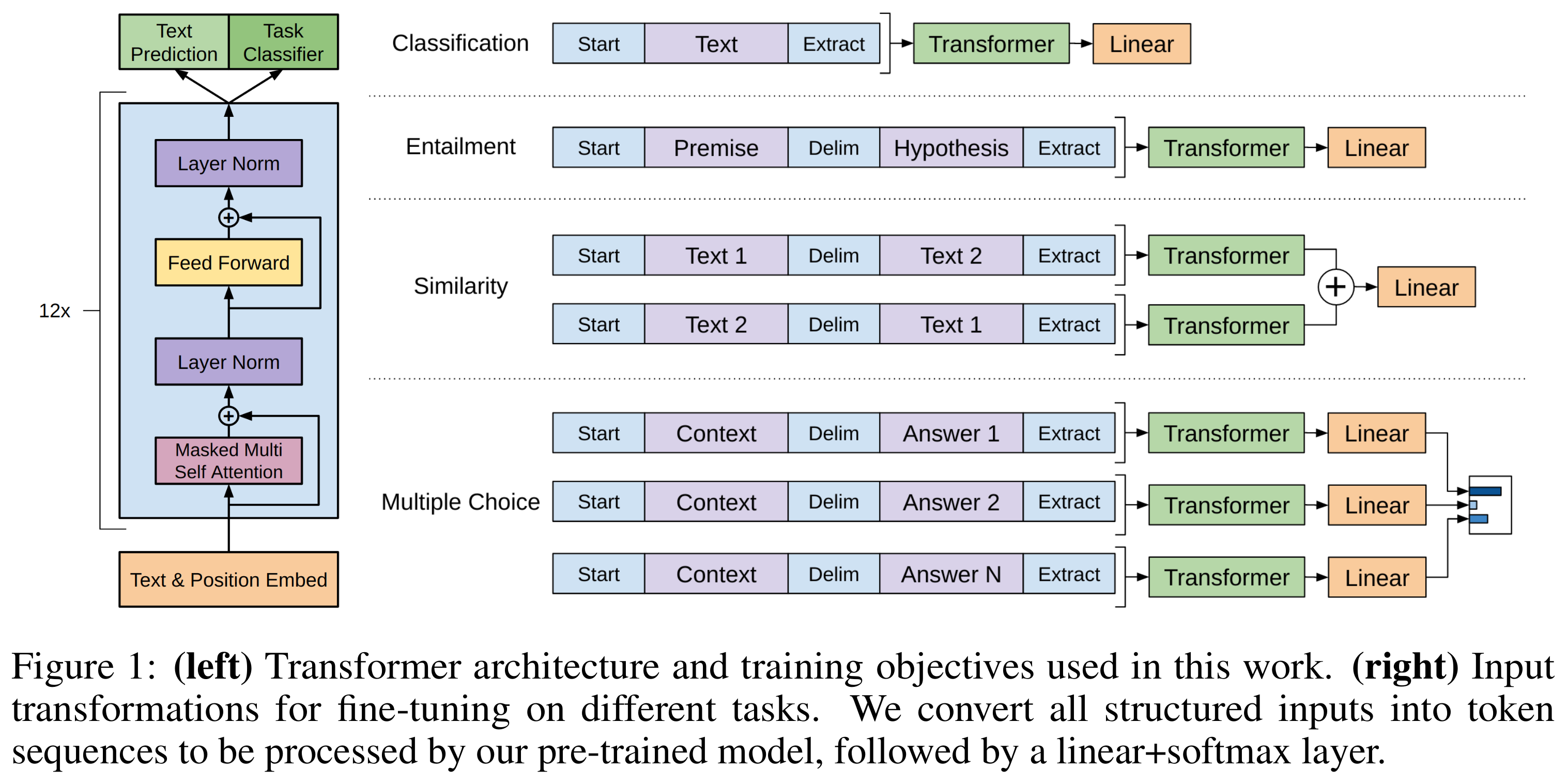The GPT-1 was first trained as an autoregressive language model. Even though it’s an autoregressive language model at inference time for producing natural languages, it’s training is non-autoregressive and it’s inference does not have to be autoregressive either. Because of this, the autoregressive GPT-1 language model can be further fine-tuned for specialized natural language processing tasks using the specialized datasets and the methods shown in the figure above.

GPT-1 is an autoregressive Transformer decoder model, whereas at the same time there was also a non-autoregressive Transformer encoder model from Google, called BERT, which was also trained with language modeling first (though the language modeling was a “masked” one) and then fine-tuned for specific natural language tasks using specialized dataset.

The autoregressive Transformer decoder model uses the masked attention mechanism where each token can only pay attention to the previous tokens, whereas the non-autoregressive Transformer encoder model uses the non-masked attention mechanism where each token can pay attention to tokens before and after itself bidirectionally. So it seems that for specialized natural language processing tasks whose inputs $X$ are always given in advance, BERT makes more sense than GPT-1. In fact, if I remember it correctly, BERT was more popular over GPT-1 and was state-of-the-art for different natural language processing tasks at that time.

It should also be noted that at that time the natural language processing tasks, such as QA, the formulation of the problem in the modeling did sound very awkward to me, even though the performance of the models, such as BERT, were very good at that time. For example, even though QA stands for question and answering, the formulation of the problem in the modeling was actually answer detection, i.e., detecting answers from the context by predicting the answer start token index and the end token index in the context, instead of answering intellectually. This kind of problems were addressed in the later GPT models.

## GPT-2

GPT-2 was the second-generation of the GPT models that OpenAI developed for natural language processing tasks in 2019. It’s the model that, for the first time, has more than one billion parameters. Thanks to the great engineering effort at OpenAI (and NVIDIA GPUs), GPT-2 demonstrated the power of large unlabeled high-quality text datasets and model capacity for solving different kind of specialized natural language processing tasks, such as QA and Translation, using language modeling in a zero-shot manner. It’s also summarized in the paper title “Language Models are Unsupervised Multitask Learners”.

In this work, OpenAI created an extremely large dataset with cautions of its quality. Because the dataset is so large, it already contains lots of “annotated” examples of for different kind of specialized natural language processing tasks. For example, the dataset might contain a piece of text “The translation of “Hello” to Chinese is “你好”.” which is a perfect example for English to Chinese machine translation. Using this dataset to train a language model, if the model has sufficient capacity and can generalize, it will perform very well on the specialized natural language processing tasks. For example, for QA task, when the context and questions were fed into GPT-2, the model no longer has to answer questions by detection, it will just answer by generating new tokens which is more similar to human behaviors. More importantly, the larger the model is, the better performance it can have for different tasks.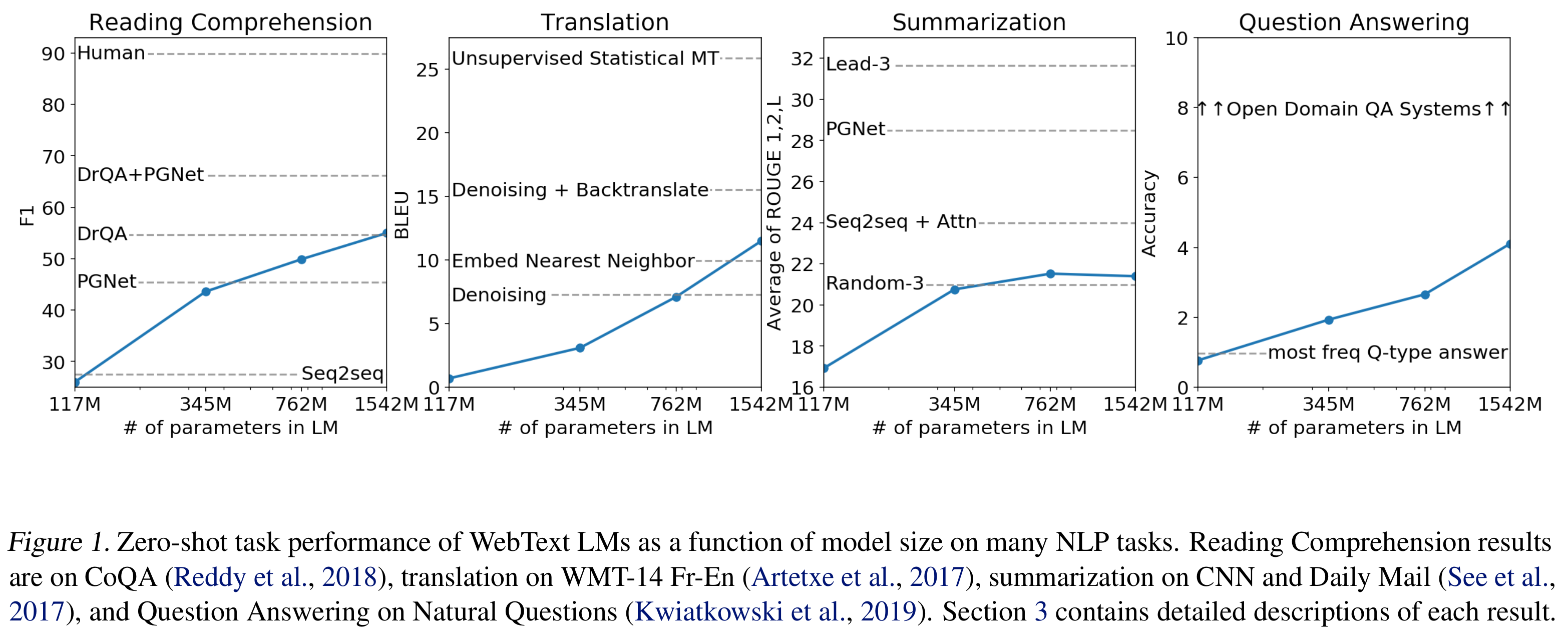In the GPT-2 work, the developers have actually started to use instructions, later known as prompt, to guide the language model to generate the desired text. For example, the question in the QA task is a natural prompt; the prompt “TL;DR:” is engineered in the training dataset for text summarization tasks. It also started to use examples to guide the language model to do desired tasks, such as adding an English to French translation example before the English sentence to be translated in order to ask the language model to do English to French translation, which becomes a very common approach in the later GPT-3 model.

## GPT-3

GPT-3 was the second-generation of the GPT models that OpenAI developed for natural language processing tasks in 2020, and it was a phenomenal one. The number of parameters of GPT-3 exceeded 175 billion, 175 times larger than GPT-2.

The GPT-3 study naturally extended the GPT-2. Its paper “Language Models are Few-Shot Learners” emphasis the “In-Context Learning” which is just by just showing a few demonstrations (few-shots) given as conditioning inputs, the language model can perform much better on specialized natural language processing tasks at inference time, without having to update the model parameters.

For example, to ask GPT-3 to do machine translation, we can describe the task first followed by showing a few demonstrations and finally gave our prompt, i.e., the sentence to be translated to GPT-3 and GPT-3 can generate the translated sentence.

In fact, we had already seen some of such evidences in the GPT-2 work mentioned previously.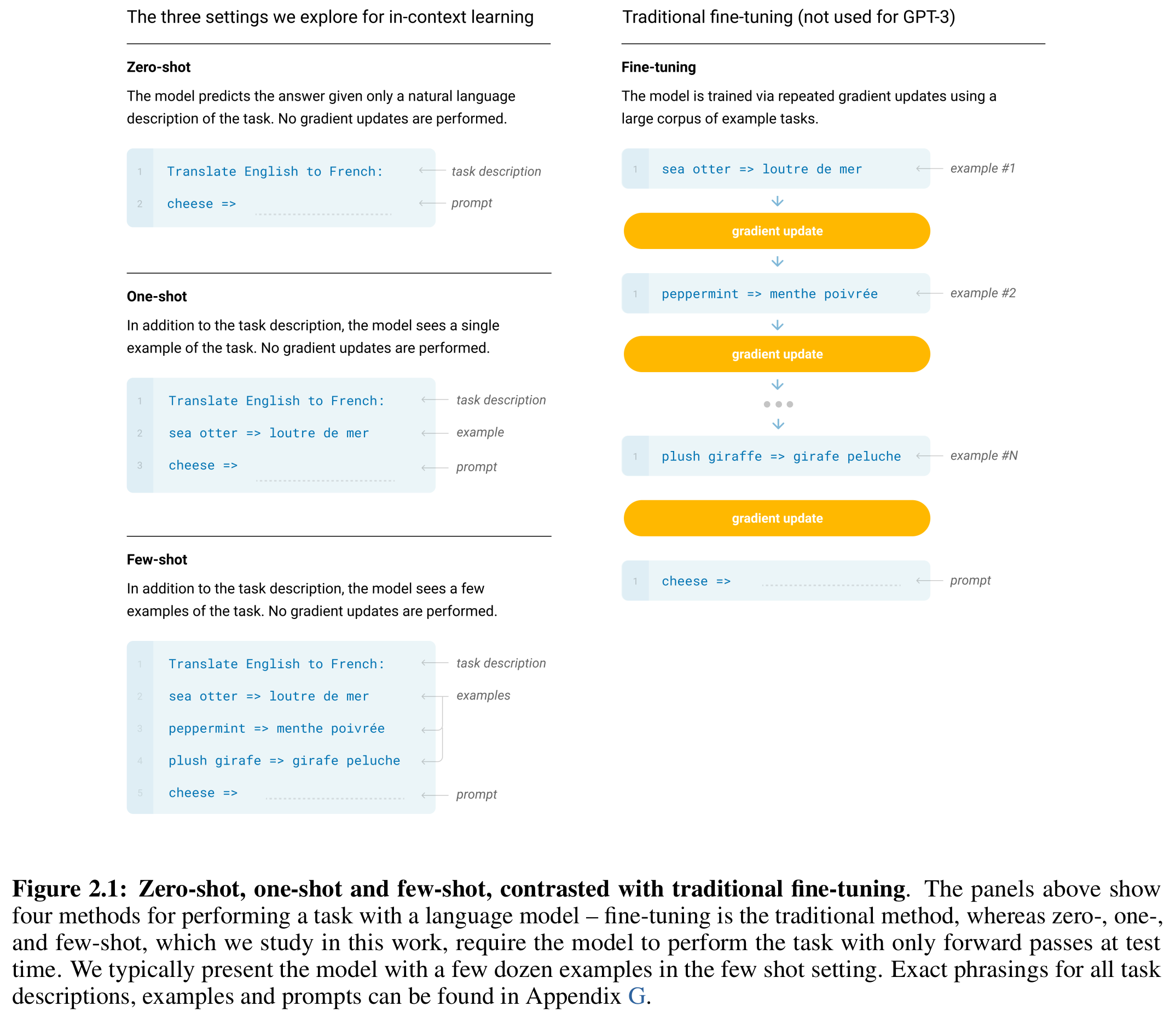In addition to these, GPT-3 used sparse attention mechanism reducing the asymptotic complexity for computing the attention matrices from $O(N^2)$ to $O(N \sqrt{N})$. This reduced the inference pressure of a 175-billion parameter model to some extent.

## InstructGPT

Even though the GPT language models so far, including GPT-3, have done an excellent job in the specialized natural language processing tasks. It’s not trained to follow human instructions, because the dataset that was used for training the GPT models previously contains less such text.

Instruction is not always a question and it might not have rich context so that the model can follow the instruction more easily.

Previously, to do the QA task in GPT-3, we would have to supply a document first, followed by the question, and some special tokens such as “A:” or “Answer:”. It’s not the natural way that human beings ask people to answer questions. Especially for some questions, we don’t need to supply a document first.

For example, if the user just asked the language model GPT-3 to “Explain the moon landing to a 6 year old in a few sentences.” without giving more context or demonstrations. GPT-3 would hardly know this is an instruction from human being. So GPT-3 can generate completions like “Explain the theory of gravity to a 6 year old.” or “Explain the big bang theory to a 6 year old.” From the perspective of natural language, those completions make sense. But from the perspective of human being, the language model did not do the job we expected.

In addition to the issue of following human instructions, the language can generate harmful or fake texts. For example, from the perspective of natural language, it’s perfectly fine for the language model to generate “I want to destroy human beings.”, especially when the large dataset used for training can never be fully and seriously examined by OpenAI developers. But this sentence is very toxic to the human readers.

InstructGPT was then developed to specifically address these issue in 2022. The training of InstructGPT involves human in the loop. The training procedure could be summarized as follows.

1. Collect demonstration data, which is a dataset that consists of prompt and the corresponding desired response demonstrated by human beings was created. A pre-trained GPT-3 model was then fine-tuned on this dataset using supervised learning. This model is denoted as the supervised fine-tuned (SFT) model.
2. Collect comparison data. Each data from the comparison dataset consists of a prompt, some sample completions from the fine-tuned model, the ranking of the completions by human beings based on their preference. A reward model (RM) that will predict a reward score given a prompt and a completion was trained using this dataset.
3. Further optimize the SFT model in a reinforcement learning environment using the reward that is generated by RM and the proximal policy optimization (PPO) algorithm. Those STF models that are further fine-tuned using PPO algorithm are denoted as PPO models.

Notice that the step 2 and 3 could be iterated in the training loop.The step 1 looks very straightforward to do to someone who is familiar with the training of language models. The step 2 and step 3 are a little bit vague from the summarized descriptions. I will go into the details of them.

In the step 2, the RM is basically another GPT-3 architecture model which consists of slightly fewer parameters. It’s pre-trained with text dataset and specialized natural language processing tasks and further fine-tuned with the comparison data collected. A regression head was attached to the GPT-3 architecture so that it can produce scalar reward score given prompt and completion inputs, just as how OpenAI was fine-tune GPT-1 for specific natural language tasks.

A ranking loss was engineered so that the RM model can learn to predict scalar reward scores using a ranking dataset that has no ground truth reward. Specifically,

\begin{aligned} \theta^{\ast} &= - \argmin_{\theta} \mathbb{E}_{(X, Y_{w}, Y_{l}) \sim P_{\text{dataset}}(X, Y_{w}, Y_{l})} \left[ \frac{1}{ {K_{X}} \choose {2} } \log \left( \sigma \left( r_{\theta}(X, Y_{w}) - r_{\theta}(X, Y_{l}) \right) \right) \right] \\ \end{aligned}

where ${K_{X}}$ is the number of completions that human beings have ranked for prompt $X$, $r_{\theta}(X, Y)$ is the scalar output of the RM for prompt $X$ and completion $Y$, $\sigma$ is the sigmoid function, $Y_{w}$ and $Y_{l}$ are a pair of completions for $X$ and $Y_{w}$ is the preferred one.

Notice that the optimization expression above is slightly different from what’s described in the InstructGPT paper “Training Language Models to Follow Instructions with Human Feedback”, because I think their expression was not correct strictly speaking and can be confusing to the readers.

In addition, I am not fully buying their explanation to the overfitting of RM if they shuffle the comparisons into one dataset instead of grouping all the comparisons for one prompt in one single batch in the InstructGPT paper. It’s very likely that they were doing the following optimizing instead without being aware of it, which screw up the training process.

\begin{aligned} \theta^{\ast} &= - \argmin_{\theta} \mathbb{E}_{(X, Y_{w}, Y_{l}) \sim P_{\text{dataset}}(X, Y_{w}, Y_{l})} \left[ \log \left( \sigma \left( r_{\theta}(X, Y_{w}) - r_{\theta}(X, Y_{l}) \right) \right) \right] \\ \end{aligned}

By fixing the loss function, they could still optimize the model the same way without overfitting even if they shuffle the comparisons into one dataset.

In the step 3, given a prompt $X$, a PPO model $\pi_{\phi}^{\text{RL}}$, where $\phi$ is the model parameter, can randomly sample a completion $Y$ with a probability of $\pi_{\phi}^{\text{RL}}(Y \vert X)$. The probability of the same prompt and completion pair being sampled from the SFT model from the step 1, $\pi_{}^{\text{SFT}}(Y \vert X)$, can also be computed. The RM can generate the reward for the prompt and completion pair $r_{\theta}(X, Y)$. Because PPO is also an language model, for any given natural language text inputs $W$, it can compute its probability $\pi_{\phi}^{\text{RL}}(W)$.

The optimization of the PPO model using PPO is to maximize the following goal that consists of three parts.

$$\phi^{\ast} = \argmax_{\phi} Q(\phi)$$

and

\begin{aligned} Q(\phi) &= \mathbb{E}_{(X, Y) \sim P_{\text{prompt}}(X) \pi_{\phi}^{\text{RL}}(Y \vert X)} \left[ r_{\theta}(X, Y) \right] - \beta \mathbb{E}_{(X, Y) \sim P_{\text{prompt}}(X) \pi_{\phi}^{\text{RL}}(Y \vert X)} \left[ \log \frac{\pi_{\phi}^{\text{RL}}(Y \vert X)}{\pi_{}^{\text{SFT}}(Y \vert X)} \right] + \gamma \mathbb{E}_{W \sim P_{\text{pretrain}}(W)} \left[ \log \left( \pi_{\phi}^{\text{RL}}(W) \right) \right] \\ \end{aligned}

where $\beta$ and $\gamma$ are the weight coefficients for different components.

The first component in the maximization goal, $\mathbb{E}_{(X, Y) \sim P_{\text{prompt}}(X) \pi_{\phi}^{\text{RL}}(Y \vert X)} \left[ r_{\theta}(X, Y) \right]$, is straightforward to understand.

\begin{aligned} \mathbb{E}_{(X, Y) \sim P_{\text{prompt}}(X) \pi_{\phi}^{\text{RL}}(Y \vert X)} \left[ r_{\theta}(X, Y) \right] &= \sum_{x \in \mathbf{\mathcal{X}} }^{} \sum_{y \in \mathbf{\mathcal{Y}} }^{} P_{\text{prompt}}(x) \pi_{\phi}^{\text{RL}}(y \vert x) r_{\theta}(x, y) \\ &= \sum_{x \in \mathbf{\mathcal{X}} }^{} P_{\text{prompt}}(x) \sum_{y \in \mathbf{\mathcal{Y}} }^{} \pi_{\phi}^{\text{RL}}(y \vert x) r_{\theta}(x, y) \\ \end{aligned}

We would like the PPO model to generate the completion $Y$ given any $X$ in a way such that the reward $r_{\theta}(X, Y)$ is maximized. This is also referred as optimizing the “policy” which is a term in reinforcement learning. This component is the major component in the optimization goal.

The second component in the maximization goal, $-\mathbb{E}_{(X, Y) \sim P_{\text{prompt}}(X) \pi_{\phi}^{\text{RL}}(Y \vert X)} \left[ \log \frac{\pi_{\phi}^{\text{RL}}(Y \vert X)}{\pi_{}^{\text{SFT}}(Y \vert X)} \right]$, is a regularization term which ensures that the policy of the PPO model does not deviate from the policy of the SFT model. In fact, this component is just a KL divergence and is called the per-token KL penalty mentioned in the paper.

\begin{aligned} \mathbb{E}_{(X, Y) \sim P_{\text{prompt}}(X) \pi_{\phi}^{\text{RL}}(Y \vert X)} \left[ \log \frac{\pi_{\phi}^{\text{RL}}(Y \vert X)}{\pi_{}^{\text{SFT}}(Y \vert X)} \right] &= \sum_{x \in \mathbf{\mathcal{X}} }^{} \sum_{y \in \mathbf{\mathcal{Y}} }^{} P_{\text{prompt}}(x) \pi_{\phi}^{\text{RL}}(y \vert x) \log \frac{\pi_{\phi}^{\text{RL}}(y \vert x)}{\pi_{}^{\text{SFT}}(y \vert x)} \\ &= \sum_{x \in \mathbf{\mathcal{X}} }^{} P_{\text{prompt}}(x) \sum_{y \in \mathbf{\mathcal{Y}} }^{} \pi_{\phi}^{\text{RL}}(y \vert x) \log \frac{\pi_{\phi}^{\text{RL}}(y \vert x)}{\pi_{}^{\text{SFT}}(y \vert x)} \\ \end{aligned}

Notice that $\sum_{y \in \mathbf{\mathcal{Y}} }^{} \pi_{\phi}^{\text{RL}}(y \vert X) \log \frac{\pi_{\phi}^{\text{RL}}(y \vert X)}{\pi_{}^{\text{SFT}}(y \vert X)}$ is just the KL-divergence between the PPO policy $\pi_{\phi}^{\text{RL}}(Y \vert X)$ and the SFT policy $\pi_{}^{\text{SFT}}(Y \vert X)$ given the prompt input $X$. In fact, I think it would be more appropriate to call it as the per-prompt KL penalty instead of the per-token penalty in the paper. Remember KL divergence is strictly non-negative and its value is 0 if and only if the two distributions are exactly the same. That’s why $-\mathbb{E}_{(X, Y) \sim P_{\text{prompt}}(X) \pi_{\phi}^{\text{RL}}(Y \vert X)} \left[ \log \frac{\pi_{\phi}^{\text{RL}}(Y \vert X)}{\pi_{}^{\text{SFT}}(Y \vert X)} \right]$ is a regularization term which ensures that the policy of the PPO model does not deviate from the policy of the SFT model. After all, SFT should already follow human instructions well, as is also demonstrated in the following figure.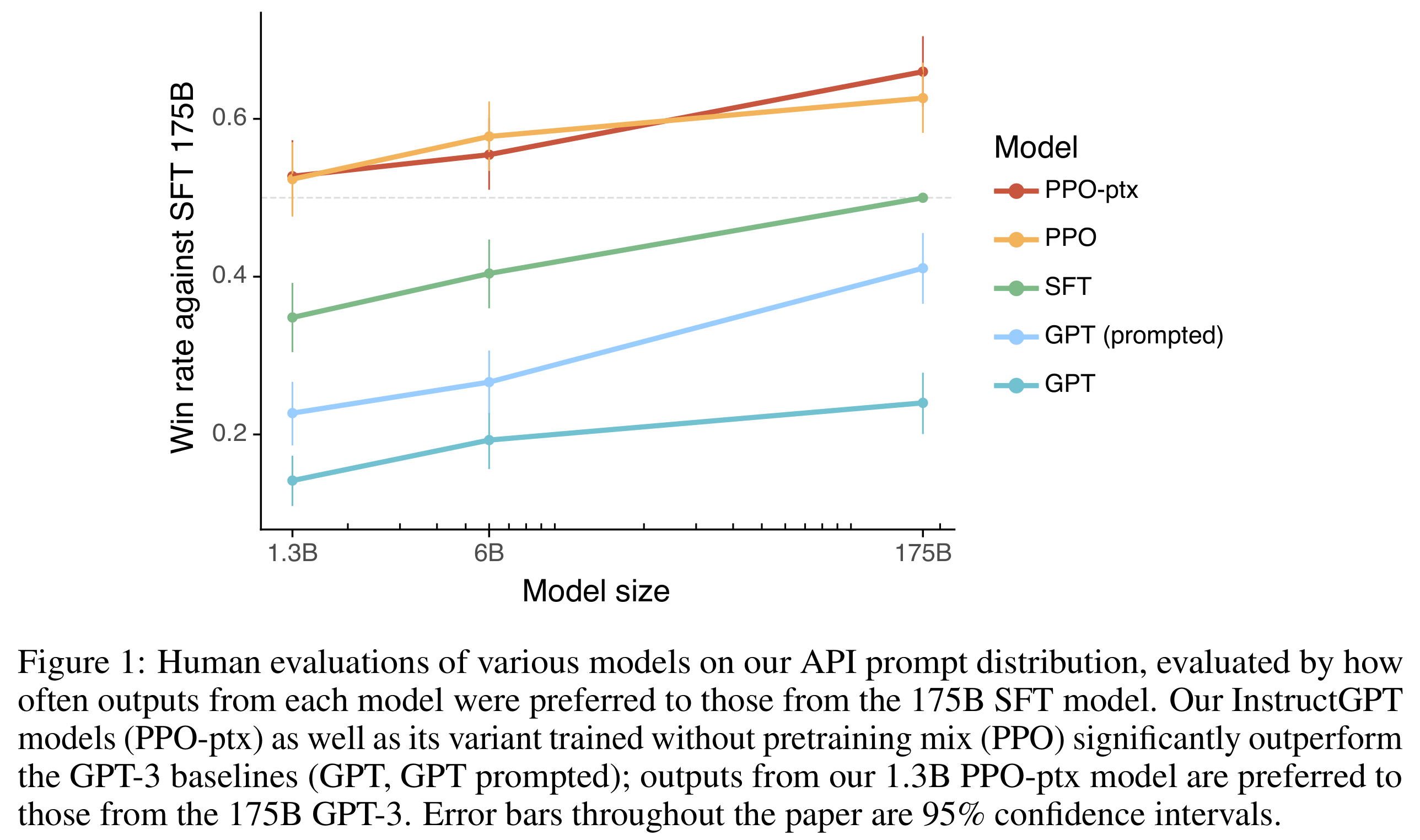The third component, $\mathbb{E}_{W \sim P_{\text{pretrain}}(W)} \left[ \log \left( \pi_{\phi}^{\text{RL}}(W) \right) \right]$, is a typical term used for language modeling. Recall that the PPO model is also an language model. Having this ensures that the PPO model generation policy would not deviate from a language model significantly in order just to maximize the reward value, especially given the RM can never be flawless.

## ChatGPT

ChatGPT was the advancement of InstructGPT that OpenAI developed in 2022. Its training process was almost the same as InstructGPT described in detail above except the demonstration data used for training the ChatGPT SFT model was different from what’s used for training the InstructGPT SFT model.As the user can tell from its name, ChatGPT is an application that allows the user to chat with AI. But the “intelligence” that ChatGPT showed in different domains was so astonishing that lots of people had started to worry losing the job because of ChatGPT. Because of this, it immediately became the most popular and the fastest user-growing application in the history.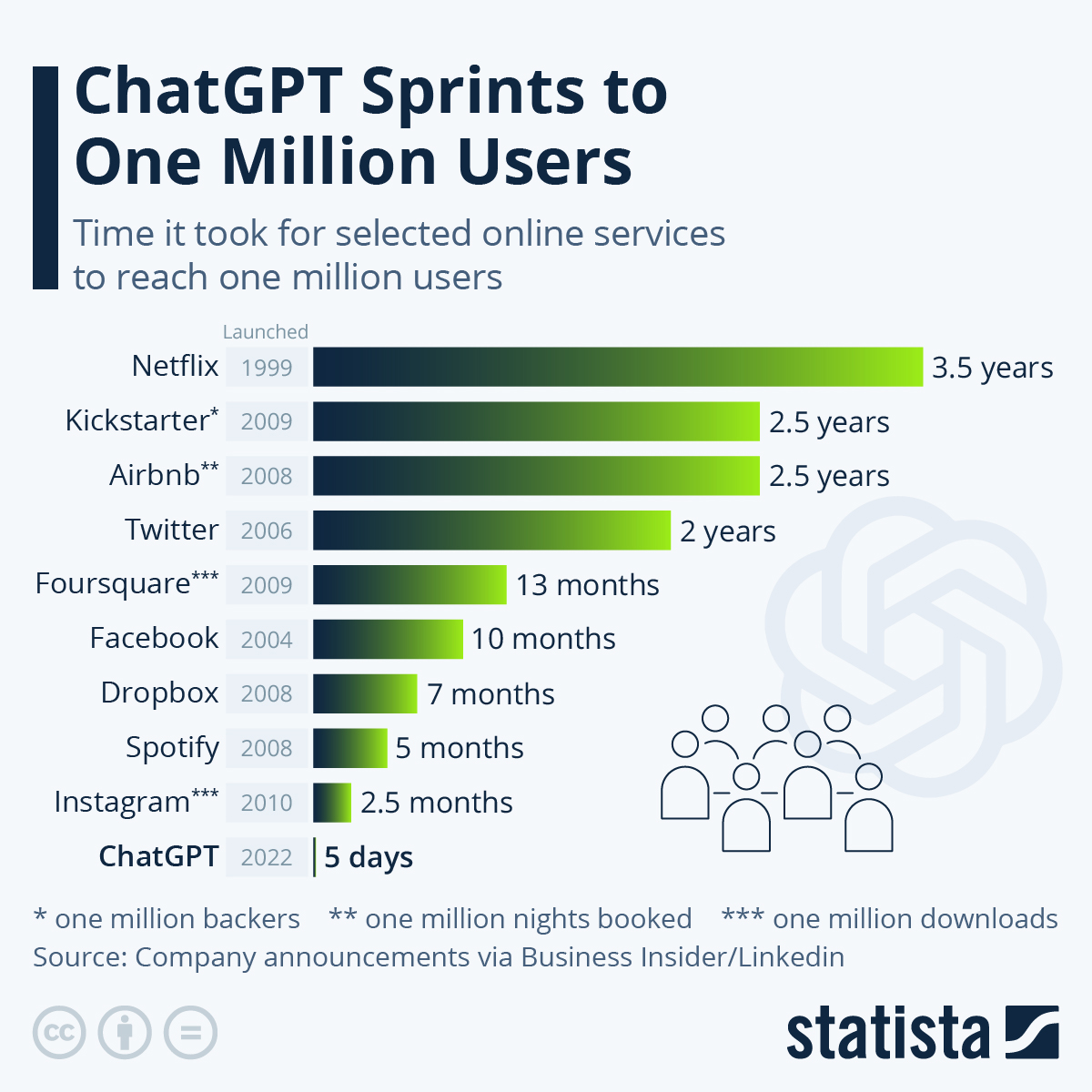What’s different between ChatGPT and InstructGPT is that InstructGPT is more like “one-shot” where the user gives one instructions and the InstructGPT does the job whereas ChatGPT allows this process to be iterative in a form of conversation without forgetting the earlier context. It allows the user to ask questions or give instructions, and refine questions or instructions. The performance of ChatGPT, as I mentioned earlier, is also very satisfying. Because of ChatGPT can help user do things and directly tell user the answer, the human being dependency on search engine might be significantly reduced. Because of this, Google has even issued a “red alert” which it has never issued before since its foundation.

Finally, let’s take a look at what’s make ChatGPT and InstructGPT different, the data. The data used for ChatGPT SFT model training are dialogues created by human beings in a special dialogue format. The responses in the dialogue can be suggested by the existing GPT models and refined by human beings. Therefore, when ChatGPT is trained, it’s still as intelligent as InstructGPT and has better user experience since the process becomes an iterative dialogue.

## Conclusions

The ideas of OpenAI GPT models are simple but extremely costly. Fortunately, as demonstrated by the results, they are also effective. The GPT model history showed OpenAI’s determination and execution in pursing the advancement of artificial general intelligence (AGI) via natural language that probably no one else had.

In the future, at least in the near future, I can imagine that the way people pursue artificial intelligence would (still) be founded on large artificial neural networks, guided by large annotated datasets, and assisted by human beings. As demonstrated by InstructGPT and ChatGPT, the language models only started to become really useful and versatile when annotated datasets and human supervisions are involved.